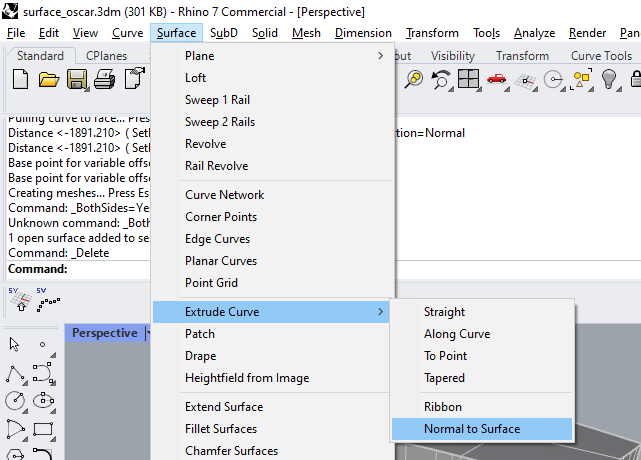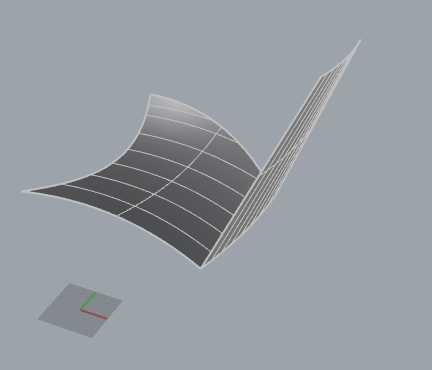# How to extrude curve normal to surface without using _Fin command

Hello.
I need to reproduce in python the command that extrudes curve normal to surface without using _Fin command.

The sample script in python using _Fin command:

``````import rhinoscriptsyntax as rs

import scriptcontext as sc
import Rhino.Geometry as rg

def RunCommand( is_interactive ):
curve = rs.GetCurveObject("Select curve")
surface = rs.GetObject("Select surface")
if curve and surface:
rs.Command('-_Fin _SelID {} _SelID {} _Direction=Normal {} _Enter _BothSides=Yes _Enter'.format(curve, surface, 500), True)

RunCommand(True)
``````